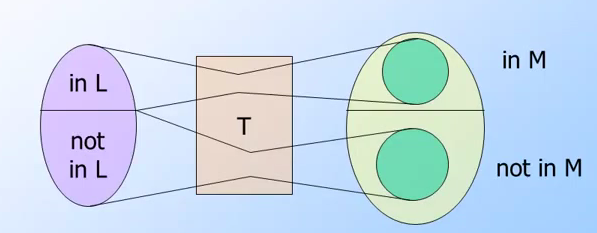## 第六週前半部分的課程內容:

###Intractable Problems

#### P類問題

T(n): 指的是輸入w長度為n的時候，該圖靈機一定會停止的


	Class P:  指的是在DTM(Deterministic Turing Machine)下，其T(n)= polynomial-time (指數時間)


DTM可以被來當成是否可以用DFA(Deterministic Finite Automata_來表示其狀態的TM(Turing Machine)． 所以其實要尋找類似的範例其實不難，只是該問題必須要是在指數時間才能解決的，課堂上提供的範例如下:

• 在CFG的 L(G) 給予一個字串w．判斷w in L(G)
• 之前有提過要快的話，必須使用CYK演算法．然後其時間複雜度為O(n^3)

#### NP類問題

	Class NP: 指在NTM(Nondeterministic Turing Machine)下，其T(n)為指數時間(polynomial-time)


##### 什麼是背包問題(Knapsack Problem)#### P = NP ?#### NP-Complete Problem

A decision problem C is NP-complete if:

• C is in NP, and
• Every problem in NP is reducible to C in polynomial time

#### Polytime Reductions

Polytime Reductions 又稱為Polynomial-time reduction，以下會解釋這種reduction 的目標與方法:

• 只要能夠找到一個方式來將所有的NP -> (Polytime Reduction) L
• 只要能夠找到Polytime Algorithm for L
• 那麼就能夠推導出其他Deterministic Polytime Algorithm給所有的NP方法:

• 先定義有L與M兩種語言
• L->(polytime reduction) M
• Transducer
• 先做Transducer將原本的資料由長度為n作轉換為x
• 透過Transducer轉換的資料長度會大於n，但是會 <= p(n)
• 將這系列動作既做 T(w) = x
• 如果w是在L裡面，那麼透過這樣轉換出來的x 必定也在M裡面．

#### Proof that Polytime Reduction “Work”!!

• 先定義有L與M兩種語言，其中:
• L有輸入w其長度為n，M有輸入x
• L有演算法p(n)， M有演算法q(x)
• L->(polytime reduction) M
• 可以透過transducer轉換出 p(n)=x
• 可以計算出M的演算法為q(x) = q(p(n))
• 全部的時間相同 L的計算時間+M的計算時間 = p(n) + q(p(n))
• 已經知道 p(n)為polytime 並且也知道 q(p(n))也為polytime
• p(n) + q(p(n)) 也是 polynomial time

### The Satisfiability Problem (SAT)

SAT是NP問題裡面的最常被提到的問題之一，而所謂的SAT就是給予一個運算式來(ex: (x+y)(-x + -y) ) 來尋找該式子為true的狀況，該x與y的組合． 這個範例裡面，解答為:

• x=0; y=1
• x=1; y=0

#### 講解Cook-Levin理論 (或稱為Cook’s Theorem)

Cook’s Theroem 準則

，Cook–Levin理論提供了很不同的思路去解決SAT是NP-Complete的問題(不過人家也花了十一年)．也就是透過演算法轉換的方式來證明這個問題是NP-Complete，而不是直接去證明這個問題是NP-Complete（from wiki)

• 已知一個NP-Complete的問題必定存在一個Algorithm，可以在Polytime解決這個NP-Complete問題．
• 如果能夠把任何演算法透過圖靈機轉換成 Boolean Formula(一個布林方程式是否存在解)
• 那麼也就能證明這個問題是NP-Complete

NTM(Nondetermistic Turing Machine) for SAT

	讓L屬於NP，而M代表NTM(Nondeterministic Turing Machine)．我們需要設計一個L，並且使得它的time-bond <= p(n) (其中n是輸入的個數)，並且 p 是 polynomial．
`

## 相關程式

• 判定錯誤的停止條件． 由於PCP是NP問題，而且又是屬於Recursively enumerable language (根據我了解的定義） 所以它無法正確的知道，何時可以停止(halt)
• 目前判斷了循環的一個case，根據我這星期研究PCP循環的case可能目前只發現兩個 (1) 解答循環 (2) 一方的長度無限制延長..